# Conjonctive rules

There are two main families of conjunctive fuzzy rules:

1. Mamdani type. The rule conclusion is a fuzzy set.
The rule is written as: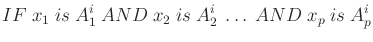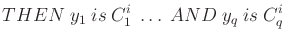where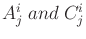are fuzzy sets defining the input and output space partitioning.

2. Takagi-Sugeno type. The rule conclusion is a crisp value.
The conclusion of the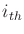rule for the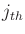output is calculated as a linear function of the input values: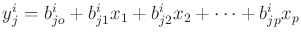, also denoted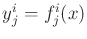.

In FisPro, for interpretability reasons, the conclusion is limited to a constant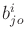.

• Rule aggregation is done in a disjunctive way for conjunctive rules, meaning that each rule opens a possible range for the output. Two operators are available to aggregate the rule conclusions: max or sum.

The rule conclusions are numerical values for a crisp output, or MF labels for a fuzzy output. They constitute a set of possible values.

The resulting levels are stored into the MuInfer array, the RuleInfer one contains the rule number corresponding to the maximum, in case of max aggregation, or the last rule number whose degree has been added, in the sum aggregation case. Both operators can be used with crisp or fuzzy output.

Note :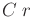the conclusion of the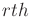rule.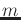the number of output MFs (fuzzy output) or the number of distinct rule conclusion values (crisp output).

The activation levels after aggregation are the following:

• max: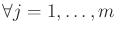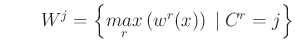• sum:

• crisp output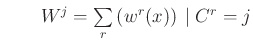• fuzzy output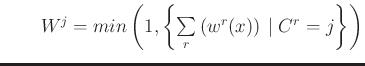• Defuzzification: This operation is required for conjunctive rules and uses aggregation results.

The inferred value,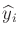for theexample, depends on the output nature and its defuzzification operators.

• crisp output
1. sugeno operator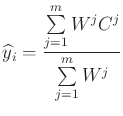(4)

2. MaxCrisp operator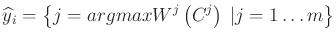• fuzzy output

Figure 10 illustrates the defuzzification possibilities for a fuzzy output.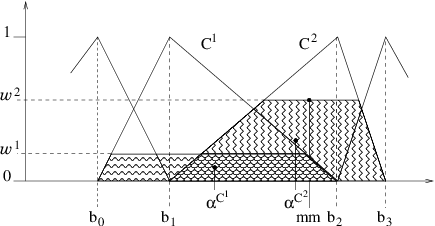1. weighted area This operator favors the interpolation between linguistic labels. The inferred output is: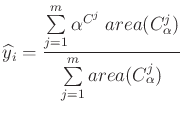(5)

whereis the number of MFs in the partition,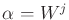is the activation level for theMF,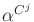is the centroid abscissa of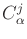, anda new MF, defined from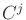as follows:2. average of maxima The output is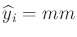(figure 10). Only the segment corresponding to the maximum activation level is considered, which means the operator mainly operates within one linguistic label.

3. sugeno It is the same formula as for a crisp output (equation 4), butnow represents the middle of theMF kernel.